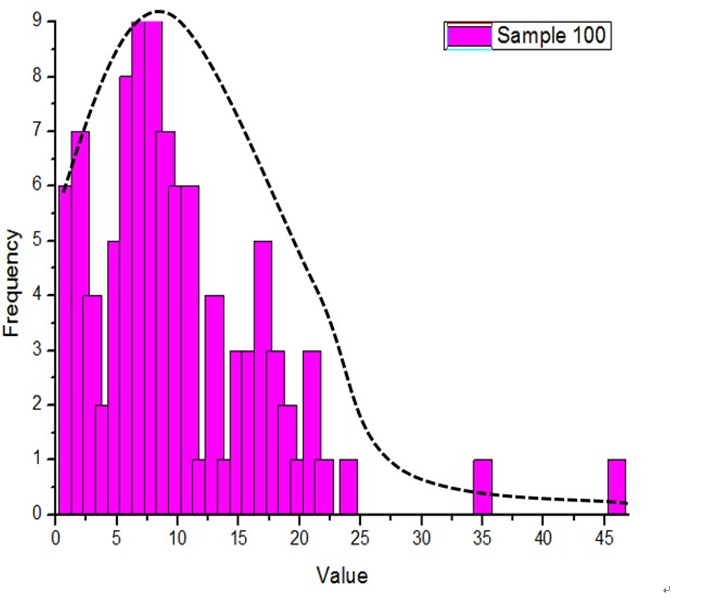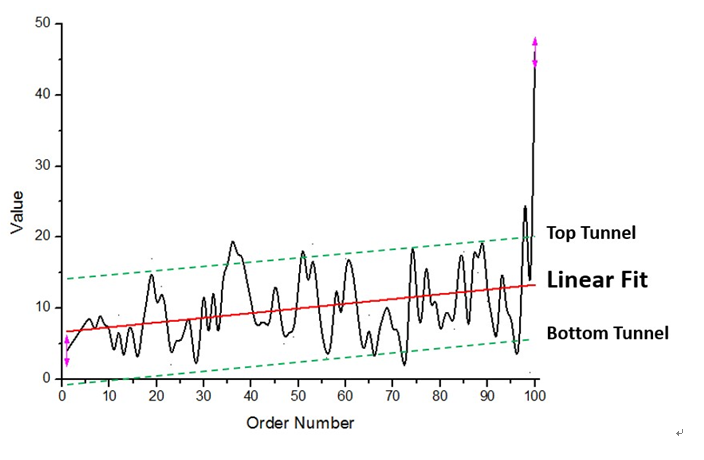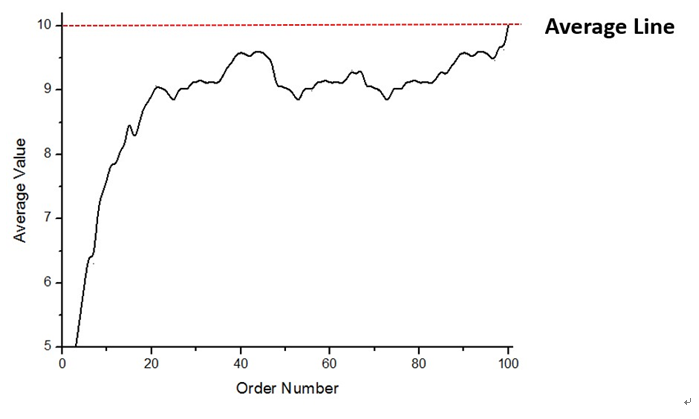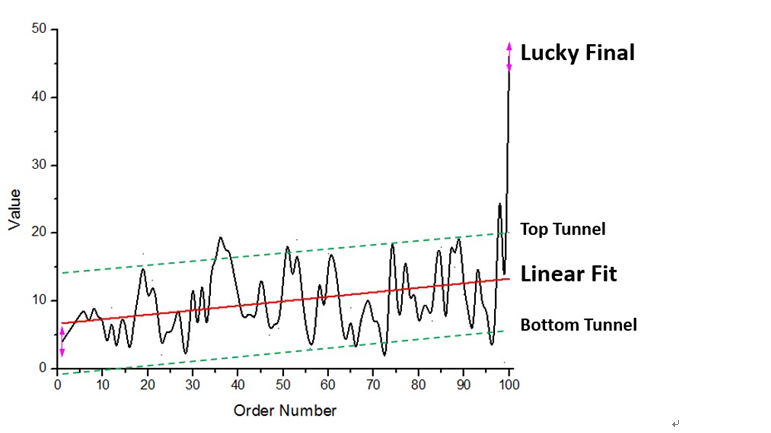### 程序员 软件开发人员

1. 钱包钱数满足截尾正态随机数分布。大致为在截尾正态分布中取随机数，并用其求和数除以总价值，获得修正因子，再用修正因子乘上所有的随机数，得到红包价值。3. 当然平均数的图还可以透露出另一个规律，那就是最后的那一个人往往容易走运抽得比较多。因为最后那一个人是钱包剩下多少就拿多少的，而之前所有人的平均数 都低于10，所以至少保证了最后一个人会高于平均值。在本样本中，98号钱包抽到35，而最后一份钱包抽到46。1. 抽到的钱大多数时候跟别人一样少，但一旦一多，就容易多很多。
2. 越是抽后面的钱包，钱越容易多。
3. 最后一个人往往容易撞大运。

1.不会有人拿不到钱

2.不会提前分完

3.钱的波动范围很大

```def weixin_divide_hongbao(money, n):
divide_table = [random.randint(1, 10000)
for x in xrange(0, n)]
sum_ = sum(divide_table)
return [x*money/sum_ for x in divide_table] ```

1.浮点数精度问题

2.边界值的处理

```int j=1;
while(j<1000)
{
int number=10;
float total=100;
float money;
double min=0.01;
double max;
int i=1;

List math=new ArrayList();
while(i<number)
{

max = total- min*(number- i);
int k = (int)((number-i)/2);
if (number -i <= 2)
{k = number -i;}
max = max/k;
money=(int)(min*100+Math.random()*(max*100-min*100+1));
money=(float)money/100;
total=total-money;
System.out.println("第"+i+"个人拿到"+money+"剩下"+total);
i++;
if(i==number)
{
System.out.println("第"+i+"个人拿到"+total+"剩下0");
}
}

System.out.println("本轮发红包中第"+(math.indexOf(Collections.max(math))+1)+"个人手气最佳");
j++;
}```

1、每个人都要能够领取到红包；

2、每个人领取到的红包金额总和=总金额；

3、每个人领取到的红包金额不等，但也不能差的太离谱，不然就没趣味；

4、算法一定要简单，不然对不起腾讯这个招牌；

N=1

N=2

N=3

……

```int j=1;
while(j<1000)
{
int number=10;
float total=100;
float money;
double min=0.01;
double max;
int i=1;

List math=new ArrayList();
while(i<number)
{

max = total- min*(number- i);
int k = (int)((number-i)/2);
if (number -i <= 2)
{k = number -i;}
max = max/k;
money=(int)(min*100+Math.random()*(max*100-min*100+1));
money=(float)money/100;
total=total-money;
System.out.println("第"+i+"个人拿到"+money+"剩下"+total);
i++;
if(i==number)
{
System.out.println("第"+i+"个人拿到"+total+"剩下0");
}
}

System.out.println("本轮发红包中第"+(math.indexOf(Collections.max(math))+1)+"个人手气最佳");
j++;
} ```

```int j=1;
while(j<1000)
{
int number=10;
float total=100;
float money;
double min=0.01;
double max;
int i=1;

List math=new ArrayList();
while(i<number)
{

max = total- min*(number- i);
int k = (int)((number-i)/2);
if (number -i <= 2)
{k = number -i;}
max = max/k;
money=(int)(min*100+Math.random()*(max*100-min*100+1));
money=(float)money/100;
total=total-money;
System.out.println("第"+i+"个人拿到"+money+"剩下"+total);
i++;
if(i==number)
{
System.out.println("第"+i+"个人拿到"+total+"剩下0");
}
}

System.out.println("本轮发红包中第"+(math.indexOf(Collections.max(math))+1)+"个人手气最佳");
j++;
}```

【译文】2015年，程序员和Unix大神们的桌面长啥样？